• 文章目录前言0. 摘要(Abstract)1. 引言(Introduction)2.... 文章通过引入对抗模型的方式缓解了低频词的训练问题。 0. 摘要(Abstract) 近年来，深度学习的应用在语言模型的提升上带来了明显的提升。然而，在实现过程中
目录前言0. 摘要(Abstract)1. 引言(Introduction)2. 背景：神经语言模型(Background: Neural Language Model)3. 主要方法(Main Method)3.1. 对抗最大似然估计(Adversarial MLE)3.2. 词嵌向量的多样性(Diversity of Embedding Vectors)4. 相关工作与讨论(Related Works and Discussions)5. 实验结果(Empirical Results)6. 总结(Conclusions)

前言
本篇文章来自Dilin Wang et al. 于2019年发表的文章：Improving Neural Language Modeling via Adversarial Training. 文章通过引入对抗模型的方式缓解了低频词的训练问题。

0. 摘要(Abstract)
近年来，深度学习的应用在语言模型的提升上带来了明显的提升。然而，在实现过程中，大规模的自然语言模型会出现过拟合的情况。在这篇文章中，我们提出了一个简单但高效的对抗训练机制对自然语言模型进行正则化处理。这项方法在训练时，于输出层的词嵌处引入了一个对抗噪音。我们展示出，在对对抗噪音的优化中存在一个简单的闭式解，因此，可以借此形式来设计一个简单有效的优化算法。理论上，我们发现了此算法可以显著的提高算法词嵌向量的多样性，以提高算法的鲁棒能力。从实验中，我们的算法将SOTA算法在PTB和Wikitext-2数据集上取得了46.01和38.07的复杂度。当我们的算法应用于机器翻译中时，可以在基于transformer模型获得一定提升，并在两项翻译数据集中均取得了BLEU的提升。
1. 引言(Introduction)
基于统计的语言模型中，机器翻译是一项测试模型的基本任务。其应用十分广阔，包括自动语音识别，机器翻译和机器视觉等。近年来，深度神经网络已经变成构建语言模型时最强劲的模型之一。而不幸的是，在训练大型RNN模型时，一项主要的挑战在于模型趋于过拟合，这样的问题通常都起源于RNN模型的高复杂度和自然语言输入的离散性。虽然许多正则化技术都用于解决此类问题，如early stop和dropout。但在现行的模型算法上，模型的训练集结果与测试集结果相差甚远，表明过拟合仍然是最常见出现的问题。
此篇文章中，我们设计了一种简单但十分有效的 极小化极大训练策略(minimax training strategy) 用于正则化。我们的想法是将一个对抗扰动加于语言模型的softmax层中的词嵌矩阵之上，并优化参数，以能最大化对抗扰动/噪音对模型的影响。值得注意的是，在我们的模型中，我们为这项扰动的优化设计了一个十分简单和高效的算法，可以让我们十分轻松的将其部署在传统模型中，而不带来额外的训练参数。
我们模型在理论上有一个十分复杂却讨好的理论特性，它可以高效促进词嵌向量的多样性，可以提升自然语言模型的泛化能力。在之前的工作中，为词嵌加入多样性这样的要求，通常通过添加额外的多样性惩罚项这种显性的方式完成。这种方式会影响优化中似然的计算，且在词表数很大时，计算成本很高。有趣的是，我们提出的这种对抗方法不需要添加额外的惩罚项，并且对比一般的正则方法，能更高效的计算。
从实验中，我们发现这种对抗算法能显著的提升在语言模型和机器翻译中SOTA算法的效果。对语言模型来说，我们建立了一个新兴的模型并在各个数据集均取得了SOTA的结果。
2. 背景：神经语言模型(Background: Neural Language Model)
一般来说，word-level的语言模型，其原理都基于条件向量的链式法则，即:
$p(x_{1:T}=\prod_{t=1}^T p(x_t|x_{1:t-1})), \tag1$
其中$x_{1:T} = [x_1, x_2, ..., x_T]$ 代表了一个长度为T的句子，$x_t \in \mathcal V$ 是第t个词，$\mathcal V$代表了字典集。在现代的自然语言模型中，条件概率$p(x_t|x_{1:t-1})$通常会被RNN所表征，其中每个时间步$t$ 的上下文表示为$x_{1:t-1}$，并且由一个隐层向量 $h_t \in \mathbb R^{d_h}$ 递归表征：
$h_t = f(x_{t-1}, h_{t-1};\bm\theta), \tag2$
其中，$f$代表了一个非线性映射，$\theta$代表可训练的参数。接下来，条件概率就可以通过一个softmax方程进行表征：
\begin{aligned} p(x_t|x_{1:t-1}; \bm\theta; \bm\omega) &= Softmax(x_t, \bm\omega, h_t) \\ &:= \frac{exp(\omega_{x_t}^\mathrm{T}h_t)}{\sum_{l=1}^{|\mathcal V|}exp(w_l^\mathrm{T}h_t)}, \tag3 \end{aligned}
其中 $\bm{\omega}={\omega_i} \subset \mathbb R^d$ 为Softmax的参数；$\omega_i$可以被看作词 $i\in\mathcal V$ 的词嵌矩阵，$h_t$ 是上下文 $x_{1:t-1}$ 的隐藏表达。内积 $\omega_{x_t}^\mathrm{T}h_t$ 衡量了词 $x_t$ 与其之前的上下文语境 $x_{1:t-1}$ 的相似度，并使用softmax函数将其转化为一个概率值。
在实现过程中，非线性映射 $f$ 由RNN的结构决定，如LSTM，GRU等。这种映射关系可能会用于另一种词嵌入向量集 $\omega_i' \in \mathbb R^{d'}$ 即
$f(x_{t-1}, h_{t-1}; \bm{\theta}) = f_{RNN}(w_{x_{t-1}}', h_{t-1}; \bm{\theta}'),$
其中$\bm\theta'$为RNN中$f_{RNN}$的权重，$\bm\theta=[\omega', \theta']$，会和 $\omega$ 一起训练。这里，$\omega_i'$是词得嵌入矩阵，它会在输入段被模型训练，也就是我们说的输入词嵌矩阵。而 $\omega_i$ 是输出词嵌矩阵。一种常用的技巧是令这两个词嵌矩阵为一个矩阵，我们称之为权重绑定(Weigh tying)，其能减少训练所需的总参数量，并带来一定的提升。
在给定一系列句子为$\{x_{1:T}^l\}_l$时，参数 $\bm\theta$ 和 $\bm\omega$ 会通过最大似然同时训练：
$\max_{\theta, \omega}\{\mathcal L(\bm\theta, \bm\omega):=\sum_{t, l}log p(x_t^l|x_{1:t-1}^l; \bm\theta, \bm\omega)\}. \tag4$
这样的优化同时训练了大量的参数$[\bm\theta, \bm\omega]$，训练同时包括了神经元中的参数，和词嵌矩阵中的参数，因此在实现中很容易引起过拟合。
3. 主要方法(Main Method)
基于在输出词嵌 $\omega_i$ 中加入对抗扰动，我们提出了一个简单的算法可以有效地减缓深度自然语言模型中的过拟合问题（如式3所示）。我们的方法简单的出奇，对比于标准的最大似然训练，几乎没有引入额外的计算量，却在一些挑战中带来了巨大的提升。我们也将输出词嵌矩阵画了出来，以展示其提升了输出词嵌向量 $\{\omega_i\}$ 的多样性。而这种多样性提升普遍能为结果的鲁棒性带来提升。
我们的想法是在输出词嵌向量 $\{\omega_i\}$ 的最大似然训练中引入一个对抗噪音：
$\max_{\bm\theta, \bm\omega} \min_{\{\delta_{j;t,l}\}} \sum_{t,l}log\ p(x_t^l | x_{1:t-1}^l; \bm\theta, \{\omega_j + \delta_{j;t, l}\}) \\ s.t. ||\delta_{j;t,l}|| \le \epsilon/2, \forall j, t, l, \tag 5$
其中 $\delta_{j;t,l}$ 是对于词 $\omega_j, j \in \mathcal V$ 的一个对抗扰动项，其位置在对第 $l$ 个句子的第 $t$ 个位置。我们使用 $||\cdot||$ 来表示L2正则项；$\epsilon$ 用于控制扰动项的大小。
上述方程有一个显著的特点：在固定模型参数 $[\bm\theta, \bm\omega]$ 下，对抗扰动项 $\bm\delta=\{\delta_{i;t,l}\}$ 有一个简单的闭式解，通过这个闭式解，我们可以推导出一个简单有效的算法（算法1）来轮流优化 $[\bm\theta, \bm\omega]$ 和 $\bm\delta$。
定义3.1. 对每个式(3)条件概率中的项 $p(x_t=1|x_{1:t-1};\bm\theta, \bm\omega) = Softmax(i, \bm\omega,h_t)$，式(5)中对抗扰动项的优化如下公式所示：
$\min_{\{\delta_j\}_{j\in \mathcal V}} \frac{exp((\omega_i + \delta_i)^T h)}{\sum_j exp((w_j + \delta_j)^Th)}\ \ s.t\ ||\delta_j|| \le \epsilon/2, \forall j \in \mathcal V.$
这就等同于只在 $\omega_i$ 上添加大小为 $\epsilon$的扰动项：
$\min_{\delta_i} \frac{exp((\omega_i + \delta_i)^Th)}{exp((\omega_i + \delta_i)^Th) + \sum_{j \not = i} exp(\omega_j^Th)}\ \ s.t\ \ |||\delta_i|| \le \epsilon,$
其又可以进一步等价于：
$\delta_i^* = \argmin_{||\delta_i|| \le \epsilon}(\omega_i + \delta_i)^Th = -\epsilon h/||h||. \tag6$
至此，我们可以得出：
\begin{aligned} AdvSoft_\epsilon(i, \omega, h) :&= \min_{||\delta_i||_2 \le \epsilon} Softmax(i, \{\omega_i + \delta_i, \bm\omega_{j \not = i}\}, h) \\ &= \frac{exp(\omega_i^Th-\epsilon||h||)}{exp(\omega_i^Th - \epsilon ||h||) + \sum_{j \not = i} exp(\omega_j^Th)} \end{aligned}
在实现过程中，我们会轮流优化参数 $[\bm\theta, \bm\omega]$ 和 $\bm\delta=\{\delta_{i:t, l}\}$. 固定 $\bm\delta$ ，模型参数 $[\bm\theta, \bm\omega]$ 使用梯度下降作为标准最大似然训练。而对 $\bm\delta$ 的训练是在固定参数 $[\bm\theta, \bm\omega]$下，使用(6)式得到的结论进行优化，基本上没有引进额外的计算量。算法 1展示了这一过程。我们的算法可以视为 $AdvSoft_\epsilon(i, \bm\omega, h)$的近似梯度下降优化，但没有对正则项 $\epsilon||h||$ 的反向传播。从实验上表明，如果对此正则项进行反向传播，反而得到的结果更差，在几个epoch后训练误差就开始离散（diverge）了。这可能是因为在 $\epsilon||h||$ 的梯度计算中，为了提高 $AdvSoft_\epsilon(i, \bm\omega, h)$, $||h||$的值被迫很大，而这与我们在实验中的设置相违背。
算法 1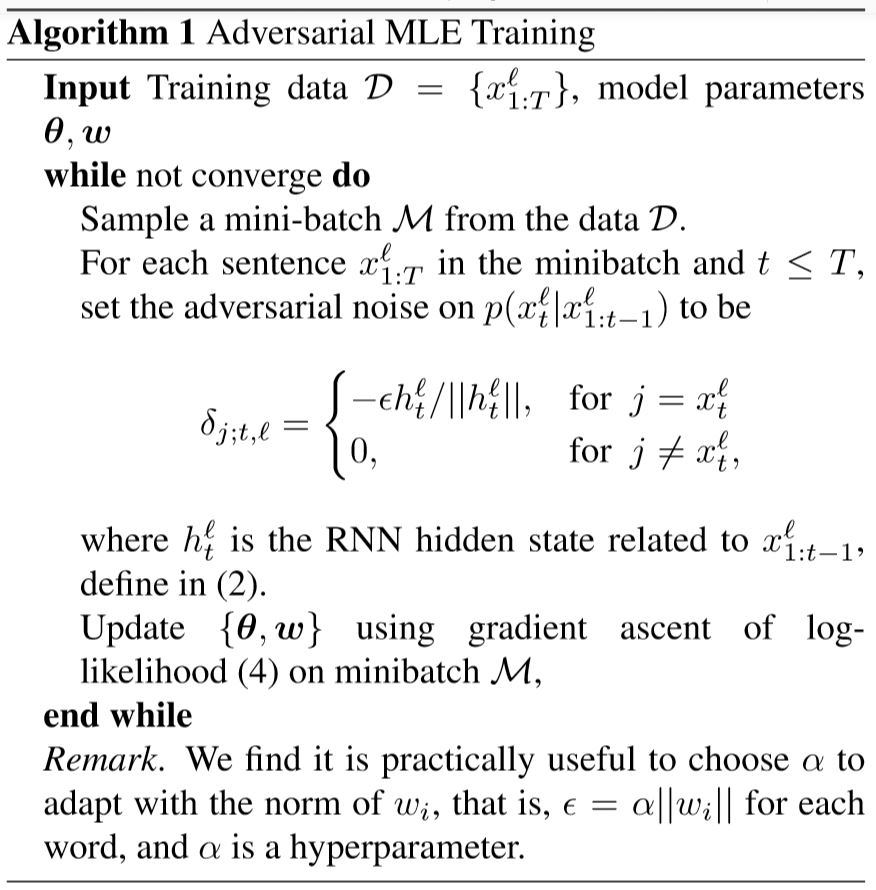3.2. 词嵌向量的多样性(Diversity of Embedding Vectors)
我们设计的对抗策略有一个十分有趣的特点：它可以被看作一个鼓励词嵌向量分布更离散的方法。我们将展示对于词嵌向量 $\omega_i$ ，一旦存在一个上下文向量 $h$ 使 $\omega_i$ 在 $AdvSoft$ 的计算中支配其他词（dominate），该算法是如何确保其分布与其他词向量离散，并保持 $\epsilon$ 的最小距离。这个简单的性质可以由对抗设置的定义来推导得出：如果在 $\omega_i$ 为原点， $\epsilon$ 为半径的球中存在一个 $\omega_j$，则 $\omega_i$ (和 $\omega_j$ )永远不会主导支配，因为处于支配地位的那个词会被对抗的噪声所惩罚。
定义 3.2. 给定一个词嵌矩阵集合 $\omega = \{\omega_i\}_{i \in \mathcal V}$，若存在一个向量 $h \in \mathbb R^d$，使 $\omega_i$ 在 $\epsilon$的对抗噪声下支配其他词， 则称 $i \in \mathcal V$ 的这个词为 $\epsilon$ -可识别，其中：
$\min_{||\delta_{i}|| \le \epsilon} (\omega_i + \delta_i)^T h = (\omega_i^Th - \epsilon||h||) > \omega_j^Th, \ \ \forall j \in \mathcal V, j \not = i.$
这时，我们可得 $AdvSoft_\epsilon(i, \bm\omega, h) \ge 1/|\mathcal V|$, 且尽管有对抗噪音，我们仍可以将 $\omega_i$ 归类为语境 $h$ 的目标词。
定理 3.3. 给定一系列词嵌向量 $\bm\omega = \{\omega_i\}_{i \in \mathcal V}$，如果词 $\omega_i$ 为 $\epsilon$ -可识别，则一定有：
$\min_{j \not =i} ||\omega_j - \omega_i|| > \epsilon,$
此时 $\omega_i$ 将与其他所有词保持最小为 $\epsilon$ 的距离。
证明 如果存在 $j \not = i$ 使 $||\omega_j - \omega_i|| \le \epsilon$ ，根据对抗优化的原理，我们可以得到：
$\omega_j^T h \ge \min_{||\delta_i|| \le \epsilon} (\omega_i + \delta_i)^Th > \omega_j^Th.$
而形成了一个对立（反证）。$\square$
注意，最大化对抗训练目标函数可以看作通过其上下文向量 $h$ 来将每个 $\omega_i$ 增强为 $\epsilon$-可识别，而也同时隐形的训练了词嵌的多样性，使其可以与其他词区分开来。我们应该注意到，在 定义3.2 中的上下文向量 $h$ 不需要在训练集出现，虽然他很可能在训练时出现。
定理 3.4. 根据式(7)中的定义，我们可以得到：
$AdvSoft_{\epsilon}(i, \bm\omega, h) \le \sigma(\Phi(i, \bm\omega, ||h||)),$
其中 $\sigma(t) = \frac{1}{1+e^{-t}}$ 是sigmoid函数，而 $\Phi(i, \bm\omega, \alpha)$ 是一个“能量方程”，它衡量了从 $\omega_i$ 到 $\omega_j$ 之间的距离，$\forall j \not= i$：
\begin{aligned} \Phi(i, \bm\omega, \alpha) &= -log\sum_{j \not= i}exp(-\alpha(||\omega_i - \omega_j|| - \epsilon)) \\ &\le \alpha \min_{j \not = i}(||\omega_i - \omega_j|| - \epsilon). \end{aligned}
证明  我们有
\begin{aligned} AdvSoft_{\epsilon}(i, \bm\omega, h) &=\frac{exp(\omega_i^Th - \epsilon||h||)}{exp(\omega_i^Th - \epsilon||h|| + \sum_{j \not= i}exp(\omega_j^Th))} \\ &= \sigma(\Psi(i, \bm\omega, h)), \end{aligned}
其中
$\Psi(i, \bm\omega, h) = -log\sum_{j \not= i}exp((\omega_j - \omega_i)^Th + \epsilon||h||)$
注意到 $(\omega_j - \omega_i) \ge -||\omega_j - \omega_i|| \cdot ||h||$ ，我们可知：
\begin{aligned} \Psi(i, \bm\omega, h) &= \log\sum_{j \not= i}exp((\omega_j - \omega_i)^Th + \epsilon||h||) \\ & \le -log\sum_{j \not= i}exp(-||\omega_j - \omega_i|| \cdot ||h|| + \epsilon||h||) \\ & = \Phi(i, \bm\omega, ||h||). \qquad \square \end{aligned}
因此，最大化 $AdvSoft_{\epsilon}(i, \bm\omega, h)$ 在我们算法中，同样也会最大化能量函数 $\Phi(i, \bm\omega\ ||h||)$ 来通过增加一个更大的惩罚项，来暴力地使 $\min_{j \not= i}(||\omega_i - \omega_j||) \ge \epsilon$。
4. 相关工作与讨论(Related Works and Discussions)
这一部分内容包括：对抗训练，直接多样化正则(Diversity Regularization)，大边界分类(Large-margin classification)，LM的其他正则技术。可以自行查阅。
5. 实验结果(Empirical Results)
由于这里我们最关心的是算法结构，实验结果可以通过开头链接，去阅读实验设置和数据集选择。
最终实验在perplexity和BLEU上都有提升，说明其设置十分合理。具体的数值请参见原始文章。
6. 总结(Conclusions)
本篇文章展示了一个对抗MLE训练策略，其在自然语言模型中可以提升词嵌空间的多样性和结果的泛化能力。这种方法可以在所有MLE基础模型上直接使用而不带来额外的训练参数和计算。通过这个方法，许多语言模型和翻译模型都得到了提升，取得了SOTA的结果。


展开全文人工智能 NLP
• 众所周知，降噪功能对耳机很重要：一是减少噪音，避免因过度放大音量对耳朵造成损害；二是过滤噪音，提高音质和通话质量。降噪可分为被动式降噪和主动式降噪，被动式降噪即物理降噪，是指利用物理特性将外部的噪声.....世平品佳诠鼎友尚2019年7月23日，致力于亚太地区市场的领先半导体元器件分销商---大联大控股宣布：旗下友尚推出基于瑞昱(Realtek)RTL8763BFR RWS的无线蓝牙耳机解决方案。众所周知，降噪功能对耳机很重要：一是减少噪音，避免因过度放大音量对耳朵造成损害；二是过滤噪音，提高音质和通话质量。降噪可分为被动式降噪和主动式降噪，被动式降噪即物理降噪，是指利用物理特性将外部的噪声与耳朵隔绝开，主要通过将耳机的头梁设计得紧一些、耳罩腔体进行声学优化、耳罩内部放入吸声材料等来实现耳机的物理隔音。被动降噪对高频率声音(如人声)的隔绝非常有效，一般可使噪声降低大约15-20dB。主动式降噪就是采用ANC、ENC、CVC、DSP等降噪技术来实现，其工作原理分别阐述如下：1、ANC(Active Noise Control)的工作原理是麦克风收集外部的环境噪音，然后系统转换为一个反相的声波加到喇叭端，最终人耳听到的声音是：环境噪音+反相的环境噪音，两种噪音叠加从而实现感官上的噪音降低。主动降噪根据拾音麦克风位置的不同，还可细分为前馈式主动降噪与反馈式主动降噪。2、ENC(Environmental Noise Cancellation)即环境降噪技术，能有效抑制90%的反向环境噪声，由此降低环境噪声最高可达35dB以上。通过双麦克风阵列，精准计算通话者说话的方位，在保护主方向目标语音的同时，去除环境中的各种干扰噪声。3、DSP(digital signal processing)主要针对高、低频噪声。工作原理是麦克风收集外部环境噪音，然后系统复制一个与外界环境噪音相等的反向声波，将噪音抵消，从而达到更好的降噪效果。DSP降噪的原理和ANC降噪相似，但DSP降噪方式正反向噪音直接在系统内部相互中和抵消。4、CVC(Clear Voice Capture)通话软件降噪技术即，主要针对通话过程中产生的回声，通过双麦克风消噪软件，提供通话的回声和环境噪音消除功能，是目前蓝牙通话耳机中最先进的降噪技术。与DSP技术和CVC技术的主要区别是：DSP技术(消除外部噪音)的主要受益方是耳机使用者本人，而CVC(消除回声)的主要受益人是通话的另一方。由大联大友尚推出的基于Realtek RTL8763BFR RWS的无线蓝牙耳机解决方案的工作原理：和传统无线蓝牙耳机相比，RWS无线蓝牙耳机将单个蓝牙装置由一个增加为两个单体耳机，并区分出主耳机和从耳机，先由手机连接主耳机，再由主耳机通过蓝牙无线方式连接从耳机，真正实现蓝牙耳机左右声道无线分离使用。不连接从耳机时，主耳机回到单声道音质。简单来说就是左右声道的耳机组成主从关系，将收到的音源传递给另外一个耳机实现真立体声的效果。采用Realtek RWS技术能够实现左右耳之间的延迟约在<<3ms，蓝牙无线耳机到手机之间的延迟约在<<100ms左右。这意味着RWS技术能够应用到大部分干扰极端严重的环境中。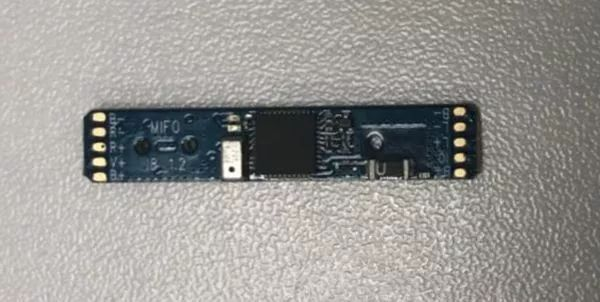图示1-大联大友尚推出基于Realtek产品的无线蓝牙耳机解决方案的展示板图▌核心技术优势Realtek RTL8763B主控芯片不仅支持蓝牙5.0、双耳通话，还支持HFP1.7、HSP1.2、A2DP1.3、AVRCP1.6、SPP1.2、PBAP1.0等多种耳机模式；Dual mode BT5.0；优异的RF性能：10dBm(typ)transmit power and receiver sensitivity to：-94.0 dBm (typ) 2M EDR；-97.0 dBm (typ) BLE；-106.5 dBm (typ) 125K BLE；支持OTA更新firmware；支持开放式SDK平台，让用户开发特色化商品；超低功耗，播放音乐时电流<8mA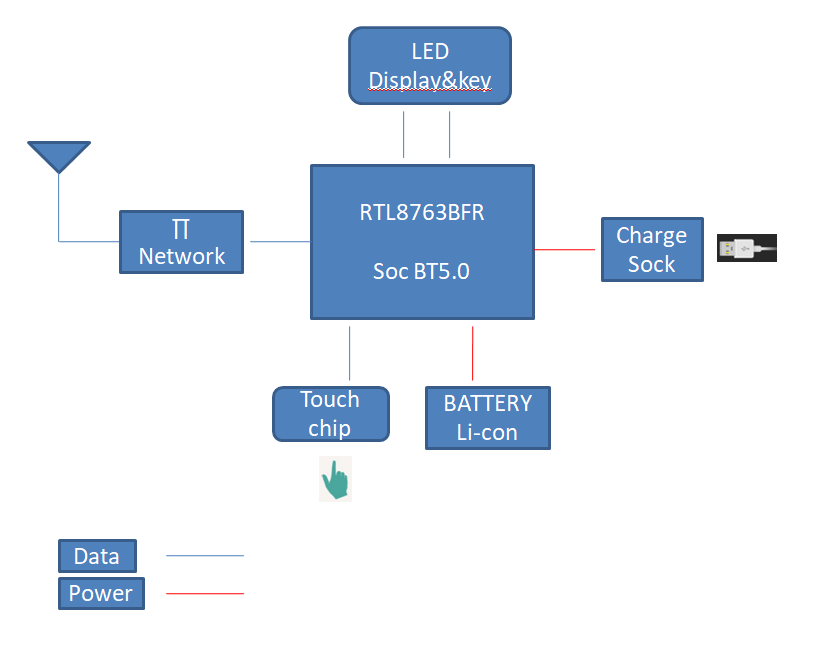图示2-大联大友尚推出基于Realtek产品的无线蓝牙耳机解决方案的方案块图▌方案规格支持双麦降噪功能(可选)；支持光感开关机；支持自动充电开关机；支持可设定化的EQ。如有任何疑问，请登陆【大大通】进行提问，超过七百位技术专家在线实时为您解答。欢迎关注大联大官方微博(@大联大)及大联大微信平台：(公众账号中搜索“大联大”或微信号wpg_holdings加关注)。展开全文• 现在的深度学习模型很容易对存在噪音的数据集过拟合，从而使得模型在测试集上的表现较差。现在比较流行的防止模型过拟合的方法：数据增强，权重衰减，dropout, batch normalization等方法并不能很好的解决这一问题。...
文章目录1 鲁棒性的损失函数2 鲁棒性的架构3 正则化4 调整损失函数5 样本选择6 元学习7 半监督学习最后的话

深度学习模型往往需要大量的标记正确的数据，而现实世界的数据集中有8%~38.5%的数据是被污染的。现在的深度学习模型很容易对存在噪音的数据集过拟合，从而使得模型在测试集上的表现较差。现在比较流行的防止模型过拟合的方法：数据增强，权重衰减，dropout, batch normalization等方法并不能很好的解决这一问题。

1 鲁棒性的损失函数
这类方法通过改编损失函数，使得模型在噪音数据集上训练后的性能与在干净的噪音数据集上的性能相当。比如分类交叉熵往往用作分类任务的损失函数，但是它本身在面对噪音数据时表现并不好。
所以有些研究者就想使用GCE, generalized cross entropy ; SCE, symmetric cross entropy来对抗噪音数据。但是这些改编的损失函数只适用于简单的情形，即任务比较简单，数据量比较少的时候。
在实做的时候，改编的损失函数往往对降低模型的性能。
2 鲁棒性的架构
这类方法中包含噪音适应层，以及用于估计噪音转移概率的专用架构。生成对抗网络也包含在这一类方法之中，我对这类方法了解有限，只知道这类方法往往难以训练而且效果并不好。
3 正则化
常用的权重衰减，dropout, batch normalization等方法足以抵抗少量噪音数据。除此之外现在预训练模型比如BERT、ELMO等也可以在一定程度上增加模型在微调阶段的鲁棒性。预训练模型主要是可以防止模型的参数在从零开始训练时受噪音数据影响而走向错误的更新方向。正则化方法和预训练方法是目前的通用的提高模型鲁棒性的方法，因为这类方法运用起来非常方便，只需要对训练过程进行小幅的修改即可，而且面对少量噪音数据时效果还不错。但是它的缺点还是比较明显的，就是面对稍多的噪音时，效果不太行。
4 调整损失函数
这类方法是指在更新参数前调整所有训练样本对损失值的影响。我们可以通过估计标签转移矩阵来调整损失值，也可以对不同的样本赋予不同的权重，也可以通对样本的类别进行调整，从而来影响最终的损失值。
5 样本选择
为了不引入错误的校正标签，很多研究者考虑直接对样本进行选择，丢弃掉疑似噪音的样本。这类方法的核心在于以怎样的规则来丢弃疑似噪音样本，很多研究者都提出了自己的丢弃方法。在这里就不一一累述了。这类方法虽然不会引入标注错误的样本，但它不可避免地会丢弃一些标注正确的样本。
6 元学习
元学习最近几年比较热门，它的主要思想是学习如何学习。元学习往往被应用于小样本学习领域，因为它可以使模型在少量样本的训练下迅速拟合。如今，有些研究者想要使用元学习来对抗标签噪音。一部分研究者想要借助元学习可以进行小样本学习的特性，用元学习训练过的模型在数据集上快速拟合，从而避免了过拟合。还有一部分研究者想学习出网络的参数更新策略和网络的损失值，这类方法往往需要有一个干净的验证集用来训练元模型，但在现实世界中，有时候很难获得干净的数据。
7 半监督学习
有些研究者想要先从少量的干净数据集上训练多个小型的网络，然后将这些网络在噪音集上的预测结果进行集成，从而筛选出可能的标签噪音数据。有些研究者通过将训练集进行分区，同一个学习算法在不同的分区上训练出不同的参数，然后对整个数据集进行标签推理，根据推理结果对相应的样本进行剔除或者标签校正。
最后的话
总的来看，目前的噪音数据处理方法大都集中在CV领域，在NLP领域相应的研究还是比较少。笔者其实更关心的是NLP领域处理噪音的方法，因为对比CV领域的数据集，NLP领域如NER，RE等基础任务的数据集噪音也是普遍存在的。笔者最近做的NER实验比较多，发现训练出来的模型在测试集上所谓的推理错误其实根本就是测试集本身的标注错误。除了人工筛选或者规则匹配的方法，笔者还没有很好的想法来找出NER数据集上的标注错误，不知道各位有没有什么好的想法？
更多算法请参考：
Learning from Noisy Labels with Deep Neural Networks: A Survey


展开全文深度学习 机器学习
• 高温条件下噪音类型及强度对抗语义干扰、图形记忆能力和手眼协调一致性的影响研究，李伟，李宏汀，本研究采用绩效任务测试、生理指标和主观评价相结合的研究方法，探究了高温条件（32℃）下噪音类型（语言噪音、交通...
• 加入噪音后的输入被称为对抗性样本。这里的对抗性的含义，与生成对抗网络GAN中的对抗是不同的。GAN中的对抗是指生成器与判别器之间的对抗，在对抗中得到性能提升。而对抗攻击是指对抗性样本对DNN的愚弄。虽然GAN中...基本概念对抗攻击是指在原始样本中加入人眼无法察觉的噪声，该噪声不影响人对预测结果的判定，但是会让深度神经网络DNN受到愚弄，产生错误的预测结果。加入噪音后的输入被称为对抗性样本。这里的对抗性的含义，与生成对抗网络GAN中的对抗是不同的。GAN中的对抗是指生成器与判别器之间的对抗，在对抗中得到性能提升。而对抗攻击是指对抗性样本对DNN的愚弄。虽然GAN中生成器的目标也是愚弄判别器，但是生成器所产生的图片是人眼能看出变化的，也就是生成器的目标是让人眼将它产生的fake图片认为是真的，同时让判别器也将fake图片认为是真的。而对抗攻击中特别强调愚弄DNN的同时，不能被人眼发觉。这是两者最大的区别。攻击分类按照攻击者具备的能力，可以分为：白盒攻击：攻击者知道模型和参数，能在攻击时与模型有交互，并观察输入输出。黑盒攻击：攻击者不知道模型和参数，能在攻击时与模型有交互，并观察输入输出。按照攻击要达到的目标，可以分为：无目标攻击（untargeted attack）：愚弄DNN时，不指定DNN误判的结果，只要让DNN将对抗性样本误判为非原来label即可。有目标攻击（targeted attack）：愚弄DNN时，指定DNN误判的结果，即让DNN将对抗性样本识别为攻击者期望的label。经典的对抗性样本生成算法（含代码）有了对抗性样本的概念和目的，那么下面就要考虑如何产生对抗性样本。对抗性样本的生成算法有很多种，最为经典的是FGSM（Fast Gradient Sign Method），也最好理解。该算法的核心思想，如下公式所示：其中，为对抗性样本，x为原始输入，为原始样本与对抗样本的差异程度，也可以理解为添加噪音的幅度限制，是将loss函数J(x,y)对x的梯度的符号取出来。可以这样理解这个公式，被攻击的DNN网络的参数，不论是白盒攻击还是黑盒攻击，都是不能改变的。那么攻击者就训练一个噪声矩阵，噪声矩阵的参数是可变的，是要在攻击过程中训练的。而loss函数通常是指导DNN网络向着负梯度方向调整参数，让其更加准确。那么攻击者训练的噪声矩阵的参数调整方向就是正梯度的方向，让DNN不准确，犯的错误更大。但是有一个前提就是不能被人眼识别出来，因此设置了一个超参数，让最终添加的噪音被限制在一定幅度。需要注意的是，噪音矩阵是每个输入都对应一个，可以看做是训练了一个输入的embedding vector。在实际应用中，通常不实用FGSM，而是用IFGSM（Iterative Fast Gradient Sign Method）。这是由于FGSM的超参数是一步到位，不方便训练。而IFGSM采用了迭代的方式，逐渐地调整噪音矩阵的参数，如下所示。其中，每次调整的步长为，下一次的对抗性样本是基于上次对抗性样本向错误方向移动产生的。下面是IFGSM的代码，包括无目标和有目标的攻击。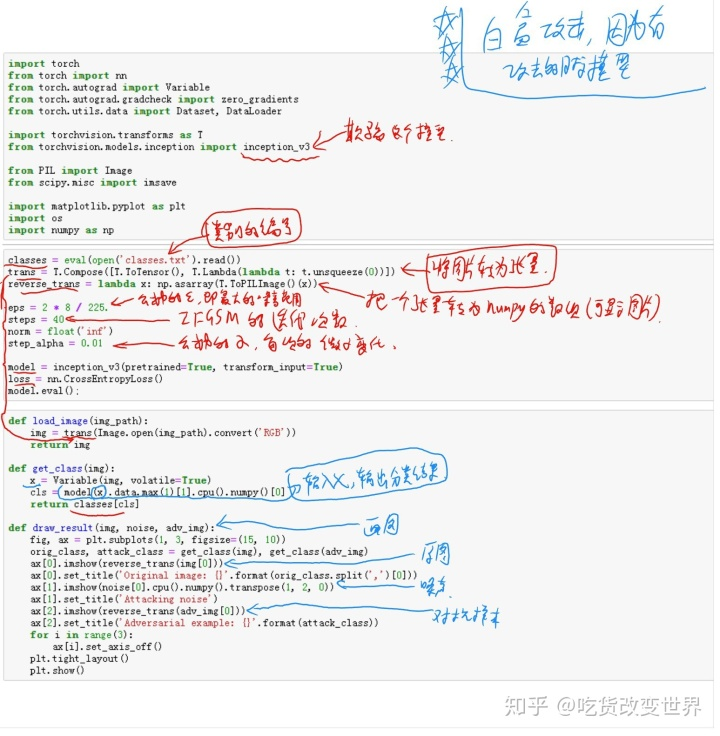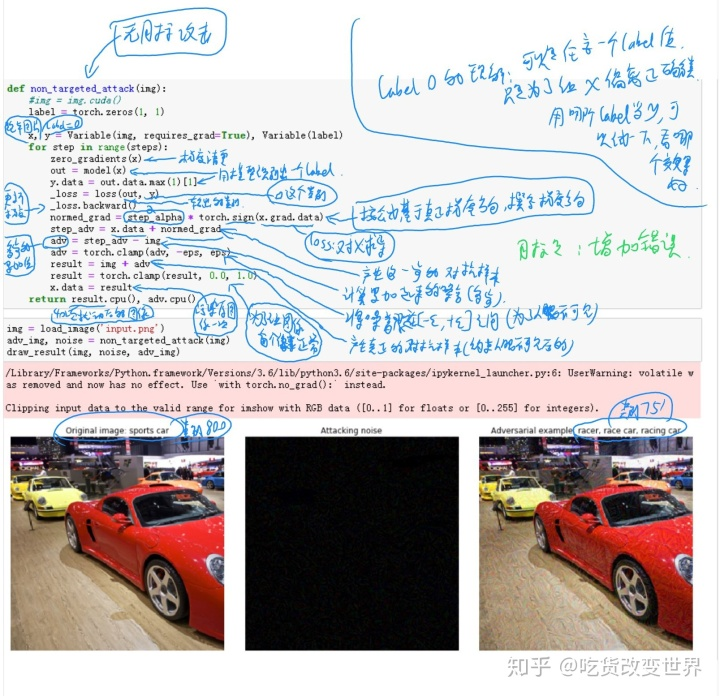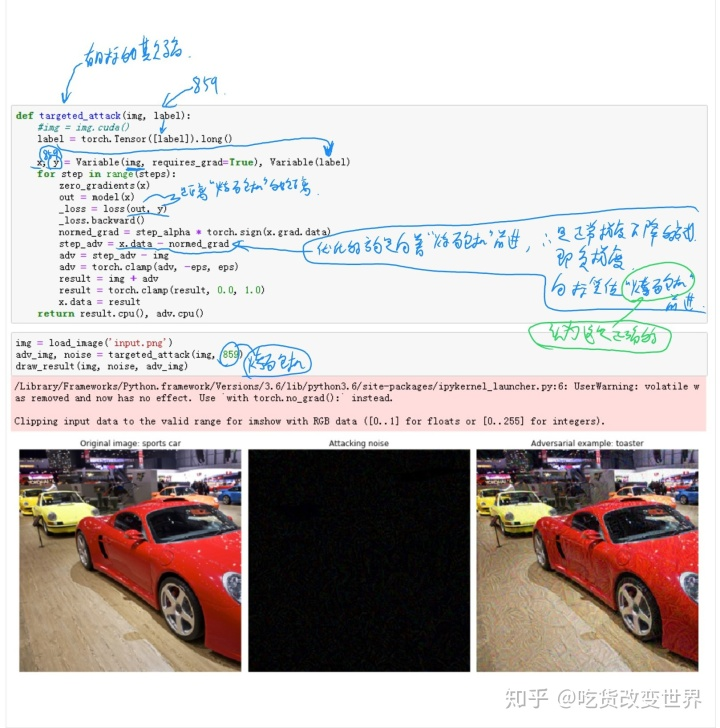经典的对抗防御方法对抗性访防御最容易想到的就是把对抗性样本加入到训练集中，让模型见多识广，就可以减少被欺骗的可能了。这种方式被称为对抗训练。由于训练数据增多了，所以对抗训练能够让模型的准确率上有所提升，且在对对抗性样本的防御上鲁棒性更好。集成对抗训练是在对抗训练的基础上采用了集成架构。即用不同的算法产生对抗性样本，构成不同的训练数据集，分别训练出若干模型，最后将这些模型进行集成使用。其缺点是，训练成本高，每次增加新的对抗性样本，都要重新训练网络。除了上述方法外，《Distillation as a Defense to Adversarial Perturbations against Deep Neural Networks 》提出了一种防御蒸馏的方法。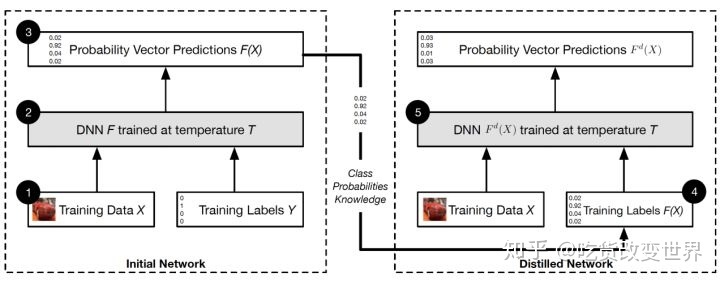其核心思想在于，将整个网络分为两部分，左侧为教师网络，右侧为学生网络。教师网络负责将特征尽可能地从输入中提取出来。即认为其输出F(X)在某种程度上体现了X的特征，视其为X的特征隐向量。在分类问题中，Label Y是（000010000）这种硬标签，而F(x)通常是（0.01，0.02,0.003,0.01,0.8,0.001）的软标签。因此，左侧教师网络就是用硬标签Y来训练网络的参数。而右边网络的输入数据同样是X，但是标签却变成了F(X)这种软标签，或者说是教师网络提取到的X的特征隐向量。F(X)将指导右侧学生网络的参数训练。从架构上就能够看出，这种方案的计算量比对抗学习小很多。因为教师网络（之前的网络）不用重新训练。下面通过公式说明这个架构为什么能够防御对抗性攻击。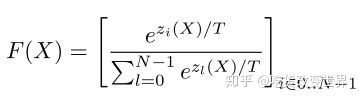教师网络的输出F(X)，并不是普通的softmax，而是增加了蒸馏温度T作为参数。下面求F(X)对X的梯度：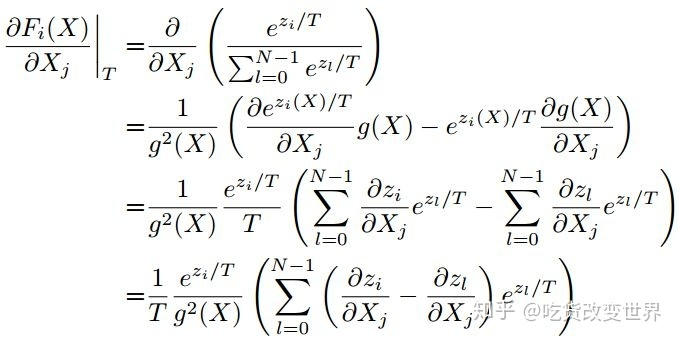可以看出，梯度的大小与T成反比。回顾一下对抗性样本产生算法FGSM：从上式看出，FGSM是在向错误方向移动。虽然FGSM的移动是固定的幅度，即改变X是固定的幅度，但是如果梯度大，那么造成的F(X)的变化就大（梯度的定义，梯度是函数值F(X)对自变量X的变化速率）。因此，当梯度大时，攻击者只需要小小地干扰一下输入X，就能让输出的判定结果变化很大。换句话说，就是梯度大时，模型对对抗性攻击是非常敏感的。因此，得出结论，通过增大T，能够让梯度减小，而梯度减小会造成模型对对抗性攻击不敏感，也就是更鲁棒了。如果攻击者要在T很大时，实现攻击效果，就需要增大，这就造成很容易被人眼发觉。最后，蒸馏是说教师网络将训练数据和硬标签中蕴含的分类知识蒸馏出来，以软标签的形式体现，而这个软标签是被蒸馏温度T控制的，因此，再基于此软标签去训练学生网络，就能够让学生网络对对抗性攻击不敏感，鲁棒性更好。
展开全文• 生成对抗网络 GAN 模型 GAN由生成器与判别器组成，需要同时训练两者，生成器通过噪音变量生成伪造的图片数据，而判别器对于输入图片来源于数据集还是伪造的图片要进行区分，生称器要尽可能的欺骗判别器（使判别器...深度学习 GAN
• ## 生成对抗网络GAN

千次阅读 2018-07-14 00:08:09
生成对抗网络GAN 理论学习 第一篇论文 Generative Adversarial Networks GAN提出者的第一篇论文下载地址 https://arxiv.org/abs/1406.2661，PDF，该论文的讲解...
• 生成对抗网络GAN（二） 语音相关@(gan)多任务对抗学习 为了获得对噪音的鲁棒性，引入多任务学习，分为三个网络： - 输入网络（绿色），用作特征提取器 - senone输出网络（红色），用作senone分类 - domain...
• 简介 生成对抗网络（Generative adversarial ...接收一个随机的噪音数据（一般服从正态分布），生生成图片，记作G(Z)。Z表示噪声数据。 判别网络 判断真实图像的输出结果 输入为真实数据X,输出X为真实图片的概率（0深度学习 神经网络
• 李宏毅——对抗模型 attack and defencemotivationattack例子如何找出特制的噪声限制how to Attack实例方法FGSM（Fast Gradient Sign Method）白盒和黑盒黑盒攻击 motivation 想在脱离实验室，实际环境中使用 在 强...
• 生成器(G)：将噪音数据生成一个想要的数据 判别器(D)：将生成器的结果进行判别， 3、代码及案例 # coding: utf-8 # ## 对抗生成网络案例 ## # # # <img src="jpg/3.png" alt="FAO" width="590" ...GAN 人工智能 深度学习
• 对抗生成网络有两个重要概念 生成器：火眼金睛，分辨出生和真实的 判别器：瞒天过海，骗过判别器 损失函数定义：一方面要让判别器分辨能力更强，另一方面要让生成器更真。 网络架构 输入层：待生成图像和...
• 文章目录gan应用gan 原理 gan应用 判别式就是将高维向低维转换 其他生成式模型复杂度很高，gan有优势 ...100维噪音向量-全连接-转置卷积。。。减少通道数量，最后输出3通道64*64 判别器就是深度借款及网络 ...
• 一 生成式对抗网络基础 ... 生成器G：将噪音数据尽量生成真实样本分布，以期望判别器D输出1 3）网络的训练阶段分为两个阶段 第一阶段：冻结生成器G，使用真实样本和生成样本训练判别器D 第二阶段：...深度学习 神经网络 tensorflow 算法 机器学习
• 本文是 GAN 在 MNIST 数据集上生成假的手写数字图片的一个实例，具体是用 ...GAN 由一个生成器和一个对抗器组成，在该任务中，生成器的输入是一堆随机生成的噪音，其输出为生成的假图片，其目标是让生成的假图片尽...
• 是时候进行一轮“噪音”了：） 这是一个重复项目，旨在进行论文“通过对抗训练增强股票走势预测”。 原始作者是冯福利，陈慧敏，何湘南，丁定，孙茂松和蔡达生。 论文链接在这里： : 项目信息 大学：西北大学 ...Python
• 但是在噪音环境及语音质量不高的情况下的，语音系统会存在识别精度下降等问题，影响用户的使用体验。为了应对低信噪比下的语音系统精度下降问题，语音增强(speech enhancement)技术的研究层出不穷。本文跟大家分享一...
• DL之AE：自编码器AutoEncoder的简介、应用、经典案例之详细攻略 ...1.2、稀疏AutoEncoder、噪音容忍AutoEncoder、卷积AutoEncoder、变分AutoEncoder→对抗AutoEncoder 自编码器AutoEncoder的经典案例 1、基础案...
• 坏人定义为G 我们通过 G 给定一个噪音X 通过学习一组参数w 生成一个G（x），转换成一个真实的分布。 这就是生成，相当于造假钱。 警察定义为D 将G(x)和真钱r 分别输入给判别网络，能判别出真假，真钱判...
• 深度学习模型容易受到输入的具体的噪音（input specific noise），定义为对抗扰动（adversarial perturbations）。 此外，这些对抗性扰动表现出跨模型的普遍性（可转移性）。这意味着相同的对抗扰动样本对不同结构...
• 为什么只要被注入的噪音抽样服从正太分布，从整体看，噪音就可以最终相互抵消？ 强差分隐私？ 数据分析？ 众包模式？大数据收集？机器学习？统计分析？ COSEC研发的适用于移动感知众包任务的交易平台是什么...
• 噪音噪音：随机标签噪音有助于对抗固有标签噪音。 学习与特征相关的标签噪声：一种渐进的方法。 健壮的早期学习：妨碍记住嘈杂的标签。 健壮的课程学习：从干净的标签检测到嘈杂的标签自我校正。 [纸] ...
• Generative Adversarial Nets 概念 提出了一种模拟二人博弈的对抗模型，对抗双方分别为： 生成模型G：尽量使生成数据与训练集...生成模型，在参数Θg条件下根据噪音z生成数据。 判别模型，判断参数Θd条件下...gan
• 为了对抗机箱噪音，电源风扇接了调速器、显卡风扇接了减速线、CPU用的是拿破仑静音版。这样一来，噪音是小了不少，但是同时问题也来了，由于fans们的转速都低了，机箱里空气对流减弱，主板温度都高达48度了。这时候...
• 但是，如果我们加入中间图片的噪音模式，则分类器认为这是一张雏菊的图片。 解决   显然，多扫视后投票和无监督预训练的策略都不能解决这个漏洞。   使用高度正则化会有所帮助，但会影响判断不含噪声图像的...
• SysML简介：SysML，全名为...但是通过在原图上添加人眼难以分辨的噪音之后，神经网络以高置信率识别右图为长臂猿。图片来自于openai.com对抗攻击威胁了现存的神经网络的应用安全。如果通过图像识别的银行，或者
• 所有声音都是由一定的频谱组成，主动降噪技术的基本原理是对已经存在的噪声进行主动对抗和消除，与传统被动防御降噪不同，主动降噪技术通过技术手段，生成一组与所要消除的噪声相位相等的反相声波，将噪音中和，达到...